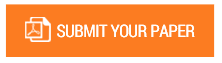Study on Simplex Method and Design & Development of Application for Solving Simplex Problem using Python

Volume/Issue : AAM – 2019

Scribd : https://bit.ly/31Zf1r9

The aim of this study is to develop application using python language which solves the simplex problem and this will help the user to understand how each step follows in simplex problem until the optimum solution is reached. Here we can optimize the objective function for minimization as well as maximization and linear constraints with n number of variables. Application developed is user friendly; python code takes input from the user in the console. Every input has a proper labelled question and the user has to enter the values of what is asked, and it shows the solution in excel with all iteration including key column and key row. The simplex algorithm using python can extend to solve real world problems like least cost formulation and maximization of profit.

Keywords : Simplex Problem, Python Application, Optimization, Excel.

#### CALL FOR PAPERS

Paper Submission Last Date
31 - May - 2022